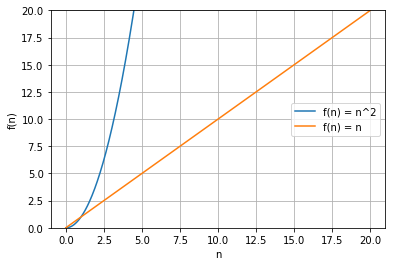# What Is Big OBig O is used to notate the ‘time and space complexity’ of algorithms. It provides us with key insights into how fast an algorithm is, without getting bogged down with details.

## Calculating the work of algorithm

Lets start with an example, consider bubblesort, which looks something like this in python:

def bubble_sort(arr):
n = len(arr)
# Traverse through all array elements
for i in range(n):
# Last i elements are already in place
for j in range(0, n-i-1):
# traverse the array from 0 to n-i-1
# Swap if the element found is greater
# than the next element
if arr[j] > arr[j+1] :
arr[j], arr[j+1] = arr[j+1], arr[j]
return arr


It loops over all the elements in the first loop, and then loops over all elements again (save the last i items), swapping any element if the next one to it is higher, to make sure the highest entry ends up in the most right position. Which means that for every item in the array, it loops over the entire array again, save a few items.

Now how can we express, in numbers now much ‘work’ this algorithm does, relative to the length of the array, $n$?

It loops over the entire array once in the first part, and then once again over every item, once more in the inner loop. For every item in that inner loop, it does a check, and then swaps 2 items if it needs to. So we could say that it does, at most $3n^2$ work. Now, it does calculate the length at the start of the function, and it does increase j every time, so thats a bit of work too. Meaning that the work could be expressed as $4 n^2 +1$. And to make this a bit more easy, i’m forgetting that after every run of the outer loop, the inner loop has to run one less time. So lets keep it at $5n^2 +1$ work. We can also call the amount of work the ‘time complexity’ of an algorithm.

Now, what if we slightly change this code, maybe optimize it a bit, then the amount of work would change. But the core of the bubble sort still remains the same, we still loop over the entire array, for each item in the array. Or what if we implement it in a different language? Having to know all details of what the compiler translates it into in bytecode would be a hassle. So lets simplify how we calculate the ‘work’ of the algorithm.

## In comes Big O

Big O provides us with a notation, which gives great insight in the efficiency of an algorithm, and how well it scales, without dealing with the details. Lets start with the calculated work of bubble sort in our previous example. ($5n^2 +1$). If we have an array of $n=100000$, then does the $+1$ really matter? At that point it has a negligible impact. And big number is what Big O is about. Because if we have an array with 7 elements, then it does not matter how efficient an algorithm sorts, because it will be done near instant regardless.

So we are left with $5n^2$. But, as mentioned previously what if we work in another language, or implement it slightly different, then do we need to run the calculation again. And we want an indicator of how efficient our algorithm is, without it being dependent on language. So in Big O, we can drop leading constants. Meaning the Big O of our bubble sort algorithm is $O(n^2)$.

## Why Big O

While Big O does remove a lot of details, it does so for a good reason. Big O tells us how well an algorithm scales. An algorithm with Big O of $O(n)$ scales a lot better than one of $O(n^2)$. Doubling the size of the input in the first case, will double the time it takes, while in the second case, the time quadruples.## When not to care about Big O

There are a lot of good reasons to care about the Big O of the algorithms you use. However if you need to sort an array of 50 items, then no matter what algorithm you use, it will be fast. Big O only starts to matter on bigger input sizes. If you need to deal with a few thousand items, then there can be a noticeable difference between $O(n \cdot log(n))$ and $O(n^2)$.

Generally speaking, the lower the Big O, the better, but don’t let that be the only fact on which to base your decision. As stated before, sometimes two algorithms with the same Big O can have a big difference in the run time. So be sure to do some profiling and see which works best for your use case.

## Closing thoughts

I left out a few steps on Big O, to keep this a bit simpler. For example, lower order bounds are also ignored in the Big O, and i didn’t go into detail on the mathematical definition of Big O, or how to prove its calculation. But i hope it did give you some insights in what Big O is, and how you can use it to decide on what algorithm to use.##### Gert de Pagter
###### Software Engineer

My interests include software development, math and magic.